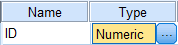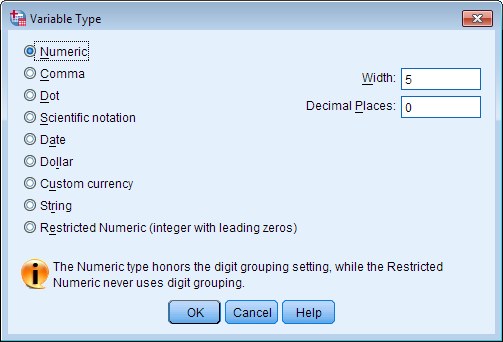# blog posts# In the continuation of the series of attractive and practical SPSS tutorials , in this post we will introduce you to the types of statistical variables and then in the form of an instructional video with an example, we will teach you how to define the questionnaire variables in SPSS.

In order for your data analysis to be accurate, it is essential that you correctly identify the type and formatting of each variable. SPSS has certain limitations so that statistical analysis cannot be performed on inappropriate types of data: for example, you will not be able to run a continuous variable as a “grouping” variable when performing a t-test. ” use.

Information for the type of each variable is displayed in the Variable View tab . Under the “Type” column, simply click on the cell associated with the variable of interest. A blue “.” Button will appear.By clicking on this option, the Type Variable Type window will appear. You can use this dialog box to define the type of variable selected and any related information (eg latitude, decimal number).The two most common types of variables you will probably see are numeric and string variables.

## Numerical

Numerical variables have numerical values ​​(in the form of a standard or a scientific sign). The failure of numeric variables in a period (for example, “.”) Appears. For example: Continuous variables that can be applied to any number in the range (such as height, weight, blood pressure,…) are considered numerical variables.

The researcher can select more or less decimal places wherever he feels it is necessary. In this case, the role setting (section) should be defined in terms of scale; See Defining Variables for more information. This particular type of numerical variable can be used to perform calculations, for example, we can calculate the mean deviation and standard height difference.

##### Example:Counts (for example, the number of free throws per game) are a numeric variable with zero decimal places. In this case, the role setting (section) should be defined in terms of scale; See Defining Variables for more information. Some mathematical calculations are valid when applied to countable variables (eg, mean and standard deviation), while some statistical methods (eg, linear regression) are not valid.

Example: Nominal deductive variables, which are numerically coded (for example, registering the gender of the subject as 1 if it is a man or 2 women) are classified as numerical variables with a decimal order of zero. In this case, the role setting (section) should be defined in Nominal format; See Defining Variables for more information. This type of numerical variable should never be used in mathematical calculations.

Example: Numerically coded batch variables (for example, a Likert item with 1 = good, 2 = better, 3 = best) are classified as numerical variables with decimal places. In this case, the role setting must be defined as Ordinal; See Defining Variables for more information. In general, this type of numerical variable should not be used in mathematical calculations. Note that some SPSS methods require that grouping variables be coded numerically. (Eg independent t-test samples, inheritance conversations for non-parametric methods, etc.)

## String in statistical variables

String variables – also called alphabetic variables or personality variables – have values ​​that are processed as text. This means that the values ​​of the string variables may include numbers, letters, or symbols.

Example: Postal codes and telephone numbers, although a combination of numbers, are usually considered as string variables because their values ​​cannot be used in calculations. For example: Each written text is a string variable, including free answers to research questions.

The next few variables are technically numerical, but show a certain formatting. If your data is stored in one of these formats, you need to set the variable type appropriately so that SPSS can interpret the variables correctly. (For example, SPSS cannot use calculations unless the variables are specifically defined as date variables.)

## Comma, comma

Numeric variables that contain commas and specify about all three locations (to the left of a decimal) and use an interval to determine decimal places. SPSS recognizes these values ​​numerically with or without commas as well as in the scientific symbol. Example: Thirty-one second: 30,000,50

Example: one million, two hundred and thirty-four thousand, five hundred and sixty-seven, and 89 thousand: 1,234,567 ٫ 89 (this number seems to be misspelled in the reference text (English))

## Point

Numerical variables that include periods (intervals) that determine about all three places and use commas to determine decimals. SPSS recognizes these values ​​numerically with or without period (interval) as well as in the scientific symbol. Example: Thirty-one-second: 30,000,50

Example: one million, two hundred and thirty-four thousand, five hundred and sixty-seven, and 89 thousand: 1,234,567 ٫ 89 (this number seems to be misspelled in the reference text (English))

Note the comma versus the dot: Commas are standard in the United States. This page from Oracle ( http://docs.oracle.com/cd/E19455-01/806-0169/overview-9/index.html ) lists countries and which symbols are usually in each of those countries Found, offers.

## scientific symbol

Numeric variables whose values ​​are denoted by the symbol E and the power of ten (the power of the tenth power). Representations (powers) can be with a D or E, with or without a sign, or with only a sign (other than D or E), be specified. Examples: .23E2, 1.23D2, 1.23E + 2, 1.23 + 2.

## Date

Numeric variables that are displayed on any standard calendar date or scheduling format. The standard format may include commas, spaces, conjunctions, periods (intervals), or points in the form of space delimiters. Example: Dates: 01/31/2013, 31/01/2013

## Dollar

Numeric variables that contain the dollar sign (ie, \$) before the numbers. A comma may be used to determine the boundaries of all three places, and an interval can be used to determine the decimal places.

Example: Thirty-three thousand dollars and 33 cents: \$ 33,000

Example: \$ 1 million and 123 cents: \$ 1,000,000 ٫ 123

## Custom Currency (Customs)

Numeric variables that are displayed in custom currency format. You must define a custom currency in the variable type window. Custom currency characters are displayed in the data editor, but cannot be used when entering data.

## Limited number (finite)

Numerical variables whose values ​​are limited to non-negative integers (in the form of a standard or scientific symbol). These values ​​are displayed by guiding the proposed zeros to the maximum of the variable digits.

Example: 00000123456 (11 digits)

## How to define questionnaire statistical variables in SPSS

In this tutorial, you will learn how to define questionnaire variables in SPSS software by giving an example.